Click to Chat

1800-1023-196

+91-120-4616500

CART 0

• 0

MY CART (5)

Use Coupon: CART20 and get 20% off on all online Study Material

ITEM
DETAILS
MRP
DISCOUNT
FINAL PRICE
Total Price: Rs.

There are no items in this cart.
Continue Shopping• Complete Physics Course - Class 11
• OFFERED PRICE: Rs. 2,968
• View Details

Electric Field

Table of Content

Introduction to Electric Field

Electric Field

Electric Force

Properties of Electric Force

Electric Field due to Multiple Charges

Physical Significance of Electric Field

Introduction to Electric Field

Coulomb’s Law states that whenever two charges are kept in a system, they exert an attractive/ repulsive force on each other. Is it necessary to have two charges, to repel or attract a charge? What if only one charge is present in the system? Scientists after working on so many experiments and fundamentals introduced the concept of electric field in order to answer above questions.

Consider a system, in which charge Q is placed at the origin (0) and the another charge q is placed at a point P. The distance between OP is ‘r’. As usual, there will be some force of attraction/ repulsion between two charges. Suppose if we remove charge q which is placed at P, from the system. Now there are two possibilities.

There is nothing at point P

There is some charge at point P

If we say there is nothing placed at point P, then why does charge q feels a force when again placed at P.? That means there is the presence of some force or field which pushes the charge q when placed at P. This proves the presence of electric field produced by electric charge Q. Whenever a charge is placed in an electric field; it experiences an electric force on it.

Electric Field

The force felt by a unit positive charge or test charge when it's kept near a charge is called Electric Field. The electric field is also defined as the region which attracts or repels a charge. The electric field is a vector quantity and it denoted by E. The standard unit of the electric field is Newton/ Coulomb or N/C.Image 1: Electric Field around charge q

If a charge q is placed in a system, then at a distance “r” at some point P, electric field is given as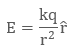where  is a unit vector and is equal to r/r. Electric field explains how electric force varies with position. The above equation can be written asThis proves electric field is actually the force between a unit positive charge (+1) also called Test Charge.

The concept of field was first proposed by Faraday, later on, it became the central concepts of physics.Image 2: Direction of electric field, radially outward for positive blue charge and radially inward for negative charge

If the electric field is positive, then its direction is radially outward, and if the electric field is negative, then its direction is radially inward. The electric field is also called Electric Field Intensity or Electric Field Strength as it determines the strength of electric field.

Example: A charge q of 2 C is kept stationary in a system. What will be the electric field produced by the charge q at a distance of 3 meters from it?

We have charge q = 2 C, and distance r = 3 m, then as we know electric field produced by charge q isTherefore charge q will produce electric field of magnitude 2 × 109 N/ C in radially outward direction.

Electric Force

We can find the force of attraction/ repulsion on a charge q placed in an electric field which is produced by charge Q, with the help of unitary method.

Let’s say electric field produced by charge Q at a distance ‘r’ is E, and we place another charge q in it. The force exerted by charge Q on charge q be “F” and is equal toSince the electric force on a unit positive charge is (+1), is E, then electric force on charge q will be q multiplied by E, that is, qE (by unitary method).Image 3: Direction of Electric Field in case of unit positive chargeImage 4: Direction of Electric Field in case of negative charge

Also standard unit of electric field is E, which is Newton/ Coulomb can be explained from the above equation by rewriting it as

E = F / q

Properties of Electric Force

Electric force “F” and electric field “E” are the interlinked quantity and show several properties which are as follows:

The charge Q which produces electric field is called Source Charge and the charge q which tests the presence and effect of electric field on it is called Test Charge.

The source charge Q must be stationary in order to make the electric field exertive when source charge Q will repel test charge q, then test charge will also try to move source charge Q and this may disturb the presence of electric field. To ensure presence of electric field we take q to be very small, or negligible with respect to Q so that electric field becomes exertive and can be represented as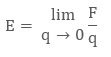The force F is proportional to q, which means F/q is a finite quantity and defines electric field

The direction of electric field for a positive charge is radially outwards from the source charge, and direction of electric field for a negative charge is radially inwards from the source charge

Electric field is same if the distance between charges are equal

The electric field on any charge depends only on distance ‘r’. Electric field depends on symmetry of charge, if we have a sphere of charge Q, then electric field will be same throughout distance r

Electric Field due to Multiple Charges

What if more than one charge is present in a system? Will the electric field be same as in the case of one charge? The case is quite similar to electric force due to multiple charges, in which we used vector method or principle of superposition to get the resultant electrostatic force. We do the same with electric fields that are take out the electric fields individually from respective charges and add them according to the principle of superposition/vector addition method.

Consider a system having charges q1 , q2, q3…… qn and let their position vectors with respect to origin be r1, r2, r3………..rn respectively

The electric field due to q1 , on a unit positive test charge at position vector r1, will be independent of those of q2 , q3 …….. qn. Thus we can apply the principle of superposition to proceed further with the process.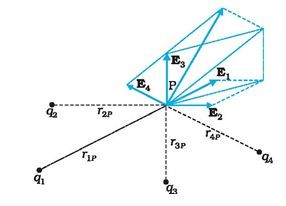Image 5: Vector diagram depicting all the electric fields

The electric field due to charge q1 is E1 and equals to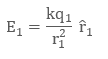The electric field due to charge q2 is E2 and equals to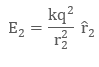The electric field due to charge q3 is E3 and equals toSimilarly, electric field due to charge qn is En and equals to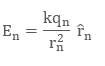Then by the principle of superposition, the net electric field E will be the vector sum of all the individual electric fields.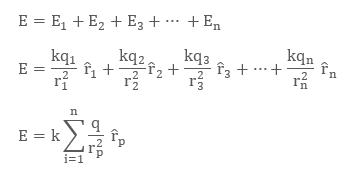The above relation can be used to find the net effective electric field when multiple charges are present in a system.

Physical Significance of Electric Field

There might be a common doubt that instead of introducing the concept of electric field, why can’t we find forces between electric charges and superposition position? The reason is electric field has a significance of its own and helps us in electrostatics, due to some of its properties like:

The concept of electric field is convenient as it tells about the electrical environment around the charges

Electric field helps in analyzing force on a unit charge at any point

Electric field is a property of system of charges and is independent of test charge

Electric field is defined at every point in space and varies accordingly

The true and useful significance of electric field is that we can apply the concept of the electric field when charges are accelerated (in motion).

Watch this Video for more reference

Electric Field### Course Features

• 101 Video Lectures
• Revision Notes
• Previous Year Papers
• Mind Map
• Study Planner
• NCERT Solutions
• Discussion Forum
• Test paper with Video Solution